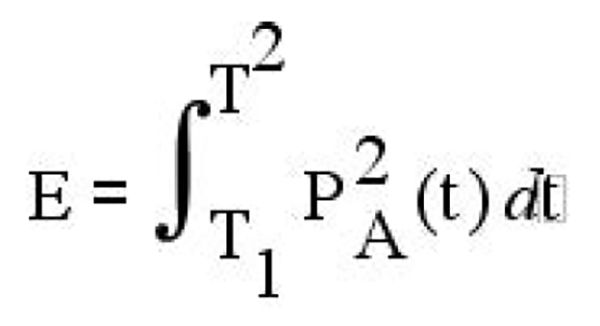# Noise Dosimetry Terminology

## Occupational Noise Measurement Glossary: Understanding Worker Noise DoseNoise Dosimetry brings with it many different options for which standard to follow and how to calculate noise dose. We've brought together some common industry terminology to help you better understand noise dose measurements. If you still have questions or have suggestions for additions to the glossary, our team of technical experts is always ready to lend a helping hand - feel free to get in touch today.

### Noise Dose (D)

It is the percentage of time a person is exposed to noise that is potentially damaging to hearing. Zero represents no exposure, and 100 represents complete exposure. At percentages over 100, the person has been exposed to noise levels that exceed their daily limit. Noise dose is calculated by dividing the actual time of exposure by the allowed time of exposure. The allowed time of exposure is determined by the Criterion Duration and by the sound level (the higher the level, the shorter the allowed time). The sound levels must be measured with A-frequency weighting and slow exponential time weighting.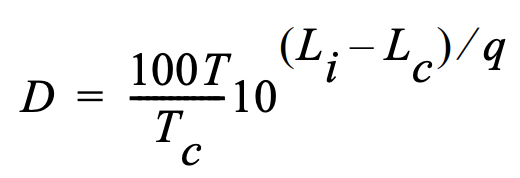where T is Measurement Duration, Tc is Criteria Time, Li is TWA, Lc is Criteria Level, q is exchange rate factor.

Standard: ANSI S12.19

### Time-Weighted Average (TWA) Noise Exposure

A time-weighted average exposure to noise over a period of time (as opposed to a noise level measured at a specific point in time). For this measurement, any noise level under the Threshold Level is ignored and the exchange rate is taken into account. When the exchange rate is 3 dB, TWA(8) is the constant sound level over an 8-hour period that produces the same amount of acoustic energy as the actual sound level, only taking into account sound above the threshold level.

### Daily Personal Noise Exposure (LEP'd/Lex,8h)

Similar to Leq in that the total sound energy is integrated over the measurement period, except that instead of averaging it over the measurement period, a reference duration of 8 hours is used. This can be thought of as the level of a constant sound over 8 hours that contains the same energy as the actual (unsteady) sound measured over the same stated measurement period. Often, the measurement period is less than 8 hours, so the actual noise exposure would be spread out (normalized) over the 8 hours.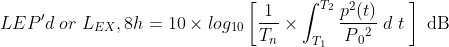OR•  𝐿𝑒𝑞 = frequency weighted (A or C), equivalent-continuous sound pressure level in dB
•  𝑇𝑛 = normalization period on criterion duration (8 hours by standard)
•  𝑇2 - 𝑇1= measurement period or Run Time

### Exchange Rate

The number of decibel increase that leads to a halving of allowable exposure time. A 3 dB increase in noise level leads to a doubling of energy (for example, a noise level of 85 dB has half as much energy as a noise level of 88 dB). An exchange rate of 3 dB is used for some standards and is the exchange rate used for Leq. However, the exchange rate is not a universally agreed upon value. Each standard, regulation, or directive will specify the exchange rate that should be used when making noise dose measurements.

For example, in OSHA regulations, the exchange rate is set at 5 dB. So, when looking at OSHA Hearing Conservation regulations, a worker may be exposed to 85 dB for 8 hours, but when the sound level is increased to 90 dB, the allowable exposure time is halved to 4 hours.

### Exposure Action Value (EAV)

A limit set on exposure to noise in an occupational environment. Expressed as a maximum time-weighted average noise exposure over a given period of time. According to OSHA, when the Exposure Action Value is exceeded, employers must develop and implement a plan to monitor worker noise exposure. The EAV is set by OSHA at 85 dBA. This means that when the 8-hour time-weighted average noise level for a worker reaches 85 dBA, the employer has to take steps to monitor exposure levels and establish a hearing conservation program.

### Threshold Level

The threshold level is applied in hearing damage risk assessment. According to the ANSI S1.25 standard, it refers to the A-weighted sound level below which the dosimeter produces little or no dose accumulation. For that reason, measured sound below this level will be disregarded. The current OSHA threshold level is 80 dB.

### Exposure Limit Value (ELV)

The maximum time-weighted average noise level an employee may be exposed to in an 8-hour day. This value varies according to which guidelines or standards are applicable.

### Recommended Exposure Limit (REL)

Limit value set by NIOSH to protect workers against the effects of excessive exposure to noise in the workplace. An 8-hour time-weighted average noise level of 85 dBA or greater for a worker is considered hazardous. NIOSH's REL assumes an exchange rate of 3 dB, or a halving of allowable exposure time for every 3 dB increase in noise level.

### Permissible Exposure Limit (PEL)

Legal limit established by OSHA in the United States for exposure to noise in the workplace. The PEL is set at 90 dBA time-weighted average over an 8-hour day. This would be considered a 100% dose. The exchange rate established by OSHA is 5 dBA. For every 5 dBA increase in noise level, the allowable exposure time is halved. Mine Safety and Health Administration (MSHA) uses the same PEL limit and exchange rate. When the PEL is exceeded, engineering or administrative controls must be put in place and hearing protection is required.

### Criterion Level (Lc)

The level of a sound, expressed in dB, which will produce a dose of 100% if continually applied for the criterion time. The criterion level is different from one standard to another. For example, the current OSHA criterion level is 90 dB. The current NIOSH criterion level is 85 dB.

Standard: ANSI S12.19

### Criterion Duration (Tc)

The time required for a continually applied sound of the selected criterion level to produce a dose of 100%. Criterion duration is typically 8 hours.

Example: If the criterion level = 90 dB and the criterion duration is 8 hours, then a sound level of 90 dB for 8 hours will produce a 100% Dose.

Standard: ANSI S12.19

### Criterion Sound Exposure (CSE)

The product of the Criterion Duration and the mean square sound pressure associated with the Criterion Sound Level when adjusted for the Exchange Rate. It is expressed in Pascals-squared seconds when the exchange rate is 3 dB, where q is the Exchange Rate Factor. See "Exchange Rate (Q), Exchange Rate Factor (q), Exposure Factor (k)".Standard: ANSI S1.25

### Sound Exposure Level (SEL, LE)

Similar to Leq in that the total sound energy is integrated over the measurement period, except that instead of averaging it over the measurement period, a reference duration of 1 second is used. It can also be thought of as the constant sound level that has the same amount of energy in one second as the total original noise event. SEL is often used as a way to compare the noise exposure from two noise events that have different durations.

Usually expressed in Pascals squared-seconds or Pascals squared-hours. P0 is the reference pressure of 20 μPa and T is the reference time of 1 second. It is then put into logarithmic form. It’s important to note that this is not an average since the reference time is not the same as the integration time.

The following equation shows that the sound pressure is squared and integrated over a specific period of time (T2-T1).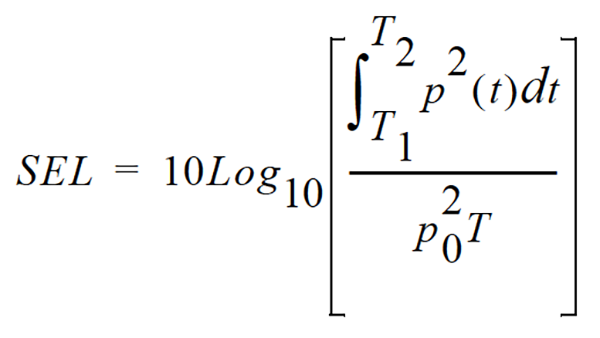Imagine comparing total energy between events with different time durations, such as:

• Dog Bark
• Automotive Passby
• Aircraft Flyover

It's convenient to have one metric to compare the total energy of individual events taken over a long period of time.

### Allowed Exposure Time (Ti)

The allowed time of exposure to sound that a constant A-weighted sound level in a chosen Criterion Level, Criterion Duration, and Exchange Rate. The equation for Ti is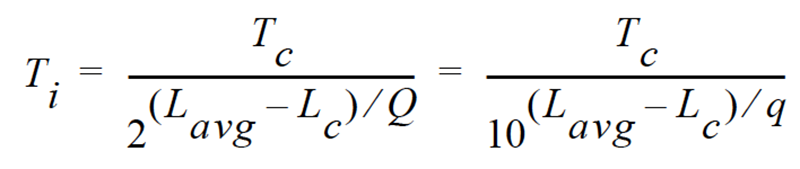where Lc is the Criterion Level, Tc is the Criterion Duration, Q is the Exchange Rate, q is the Exchange Rate Factor and Lavg is the Average Sound Level. Example: If Lc = 90, Tc = 8, Q = 3 and Lavg= 95 then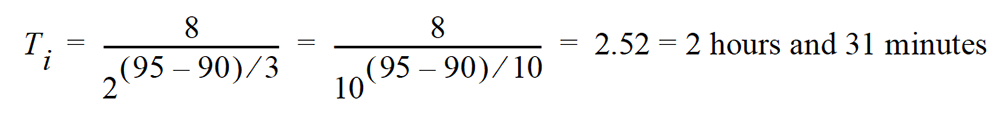This means that if a person is in this area for 2 hours and 31 minutes he will have accumulated a Noise Dose of 100%.

Standard: ANSI S12.19.

See "Exchange Rate (Q), Exchange Rate Factor (q), Exposure Factor (k)"

### Average Sound Level (Lavg)

The logarithmic average of the sound during a Measurement Duration (specific time period), using the chosen Exchange Rate Factor. Exposure to this sound level over the period would result in the same noise dose and the actual (unsteady) sound levels. If the Measurement Duration is the same as the Criterion Duration, then Lavg=LTWA(LC) where the Measurement Duration (specified time period) is T=T2-T1 and q is the Exchange Rate Factor. Only sound levels  above the Threshold Level are included in the integral. Standard: ANSI S12.19 above the Threshold Level are included in the integral.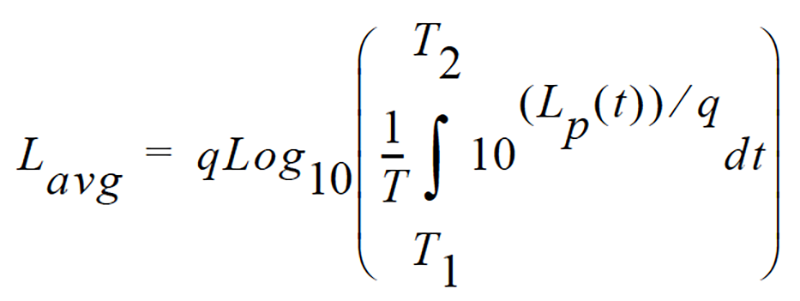Standard: ANSI S12.19

### Department of Defense Level (LDOD)

The Average Sound Level calculated in accordance with Department of Defense Exchange Rate and Threshold Level. See "Average Sound Level (Lavg)".

### Dose

See "Noise Dose (D)".

### Eight Hour Time-Weighted Average Sound Level (L TWA(8))

It is the constant sound level that would expose a person to the same Noise Dose as the actual (unsteady) sound levels. According to the ANSI S12.19 standard, the equation for it is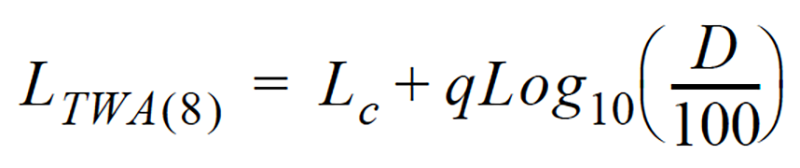NOTE: This definition applies only for a Criterion Duration of 8 hours.

### Exchange Rate (Q), Exchange Rate Factor (q), Exposure Factor (k)

It is defined in ANSI S1.25 as “the change in sound level corresponding to a doubling or halving of the duration of a sound level while a constant percentage of criterion exposure is maintained.” The rate and the factors are given in the table below.

Exchange Rate,Q Exchange Rate Factor, q Exposure Factor, k
3.01 101
413.29 .75
516.61 .60
6.0220.50

Standard: ANSI S12.19

### Measurement Duration (T)

The time period of measurement. It applies to hearing damage risk and is generally expressed in hours.

Standard: ANSI S12.19

### OSHA Level (LOSHA)

The Average Sound Level calculated in accordance with the Occupational Safety and Health Administration Exchange Rate and Threshold Level.

### Projected Noise Dose

Projected Noise Dose is the Noise Dose expected if the current level of noise exposure continues for the full Criterion Duration period.

### Single Event Noise Exposure Level (SENEL, LAX)

The Single Event Noise Exposure Level is the total sound energy over a specific period. It is a special form of the Sound Exposure Level where the time period is defined as the start and end times of a noise event such as the passing of an aircraft or automobile.

### Sound Exposure (E)

Sound Exposure is the total sound energy of the actual sound during a specific time period. It is expressed in Pascals squared seconds, or Pascals-squared hours. The following formula describes exposure in terms of E, where pA is the sound pressure and T2 - T1 is the Measurement Duration (specific time period).